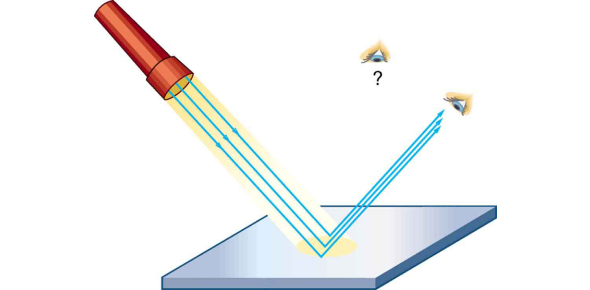# Quiz: Light And Reflection (Sph3up)

12 Questions | Attempts: 1581
ShareSettingsSample quiz for optics unit

• 1.
Calculate the index of refraction for an object in which light travels at 1.97 x 108 m/s.
• A.

1.52 x 108 m/s

• B.

0.66 m/s

• C.

1.52 m/s

• D.

1.95

• E.

1.52

• 2.
A light ray has an angle of incidence of 34º. The reflected ray will make what angle with the reflecting surface?
• A.

• B.

34º

• C.

56º

• D.

66º

• E.

74º

• 3.
A YAG laser has a frequency of 2.8 ´ 1014 Hz. What is the wavelength of the light it produces?
• A.

1.2 x 10–23 m

• B.

1.1 x 10–6 m

• C.

1.2 x 10–2 m

• D.

9.4 x 105 m

• E.

8.5 x 10–22 m

• 4.
An student is located 1.8 m from a plane mirror. How far is the image from the student?
• A.

0 m

• B.

0.9 m

• C.

1.8 m

• D.

2.7 m

• E.

3.6 m

• 5.
The image in a plane mirror is
• A.

Upright and virtual

• B.

Laterally inverted and virtual

• C.

Inverted and virtual

• D.

Two of A, B, and C

• E.

All of A, B, and C

• 6.
An object is placed between F and C of a concave mirror.  The image will be located:
• A.

Beyond C

• B.

Between F and C

• C.

Between F and the vertex

• D.

Behind the mirror

• E.

Not enough information provided

• 7.
An object is located at the focal point for a convex mirror.  The image produced will be
• A.

Inverted and real

• B.

Upright and real

• C.

Inverted and virtual

• D.

Upright and virtual

• E.

No image will be produced

• 8.
Objects placed between F and the vertex of a concave mirror will produce images that are:
• A.

Larger than the object

• B.

Virtual

• C.

Upright

• D.

Two of A, B and C

• E.

All of A, B and C

• 9.
Sometimes rays of light approaching curved mirrors do not all meet at the focal point.  This is due to a phenomenon known as:
• A.

Refraction

• B.

Chromatic abberation

• C.

Spherical abberation

• D.

Dispersion

• E.

Diffraction

• 10.
To properly use a shaving mirror (vanity mirror) your face must be located:
• A.

On the focal point

• B.

Between the focal point and the mirror

• C.

On the centre of curvature

• D.

Beyond the centre of curvature

• E.

Between the centre of curvature and the focal point

• 11.
When driving at night, headlights of cars viewed in the rearview mirror can be a distraction for the driver.  This helps reduce the amount of light entering your eyes because
• A.

Only a small percentage of light is reflected off the glass surface

• B.

Only a small percentage of light is reflected off the silvered surface

• C.

The light reflecting off the glass is sent away from the driver's eyes

• D.

The light entering the eyes are of a different colour

• E.

Light entering the glass is absorbed by the glass to make it dimmer

• 12.
A convex mirror of focal length 10 cm is used to create an image of a duck one-third its original height.  The duck must be located:
• A.

5 cm from the mirror

• B.

10 cm from the mirror

• C.

15 cm from the mirror

• D.

20 cm from the mirror

• E.

30 cm from the mirror

## Related TopicsBack to top
×

Wait!
Here's an interesting quiz for you.